Ex 8.1

Chapter 8 Class 12 Application of Integrals
Serial order wise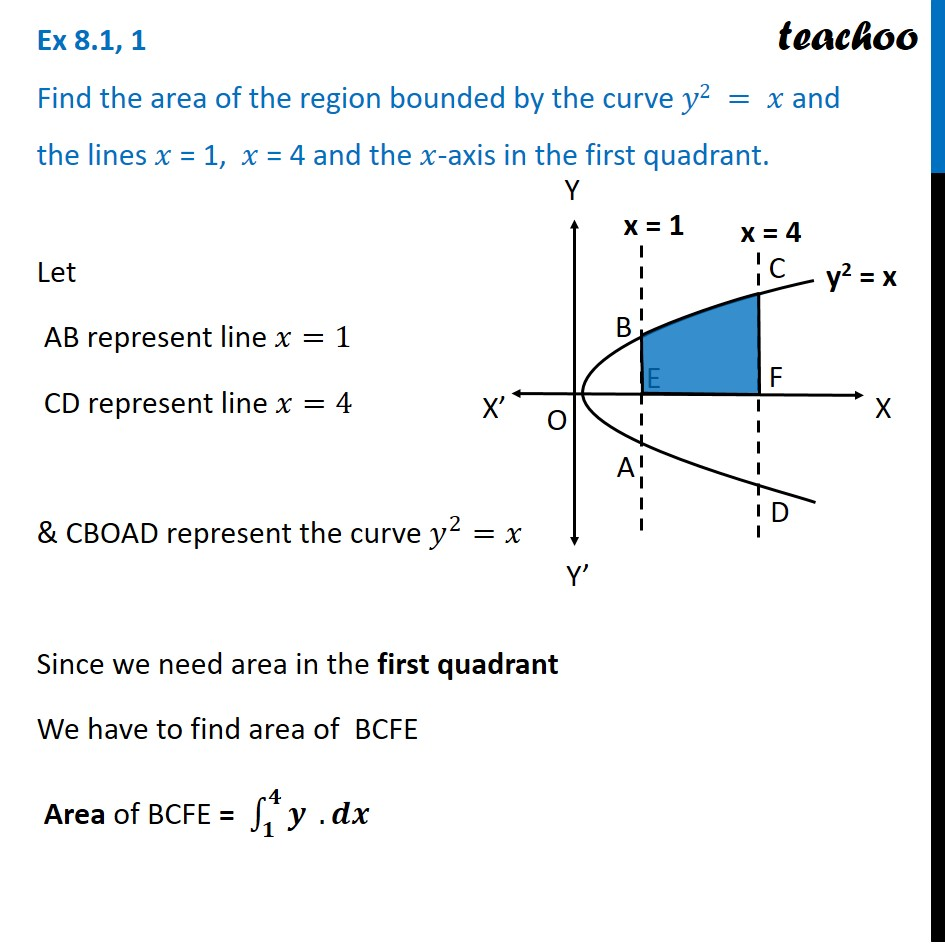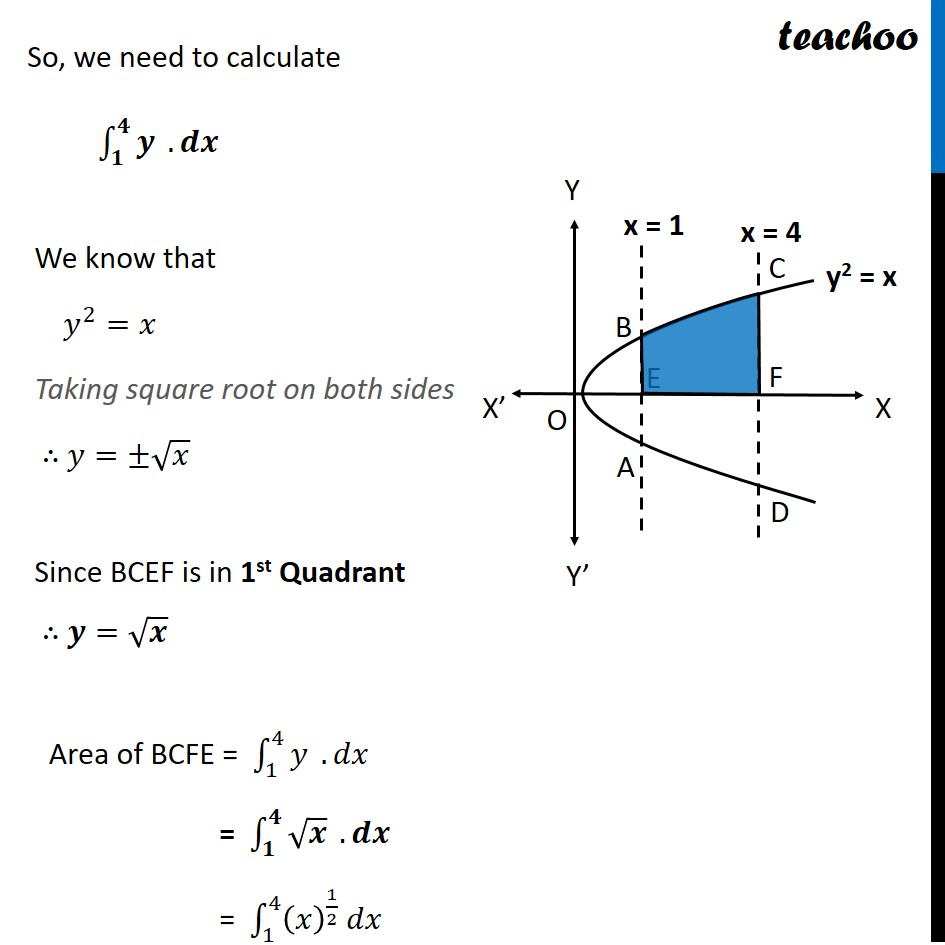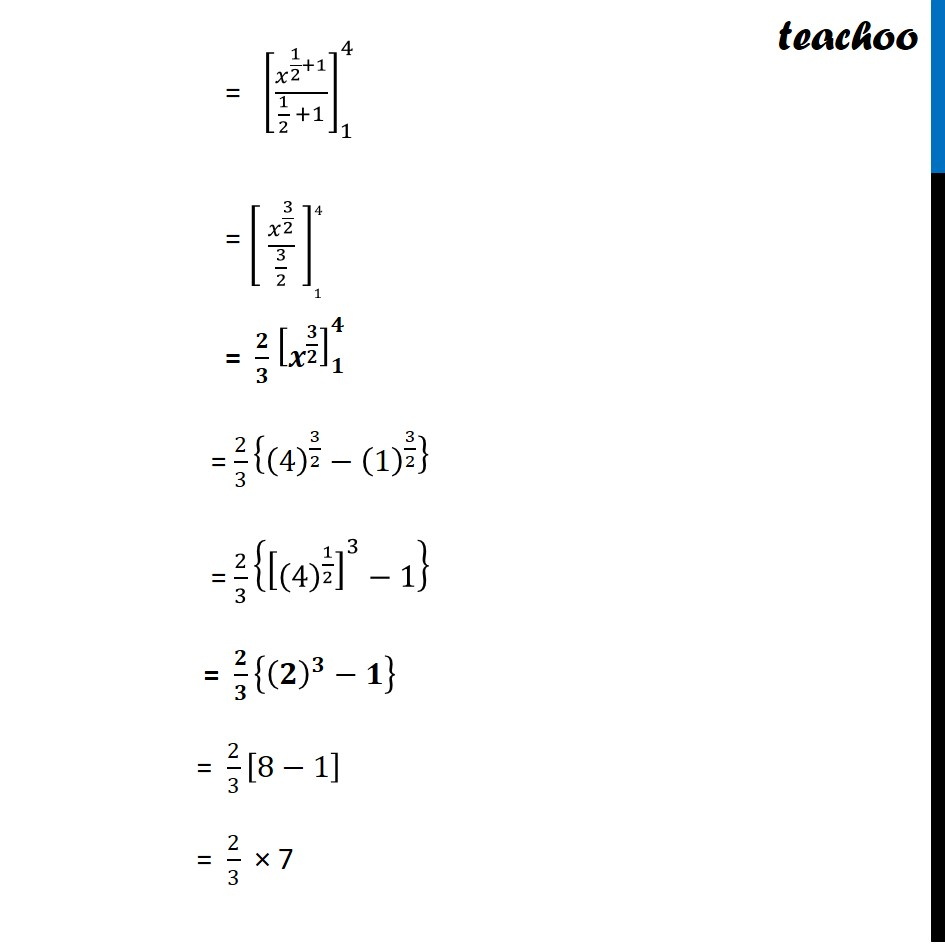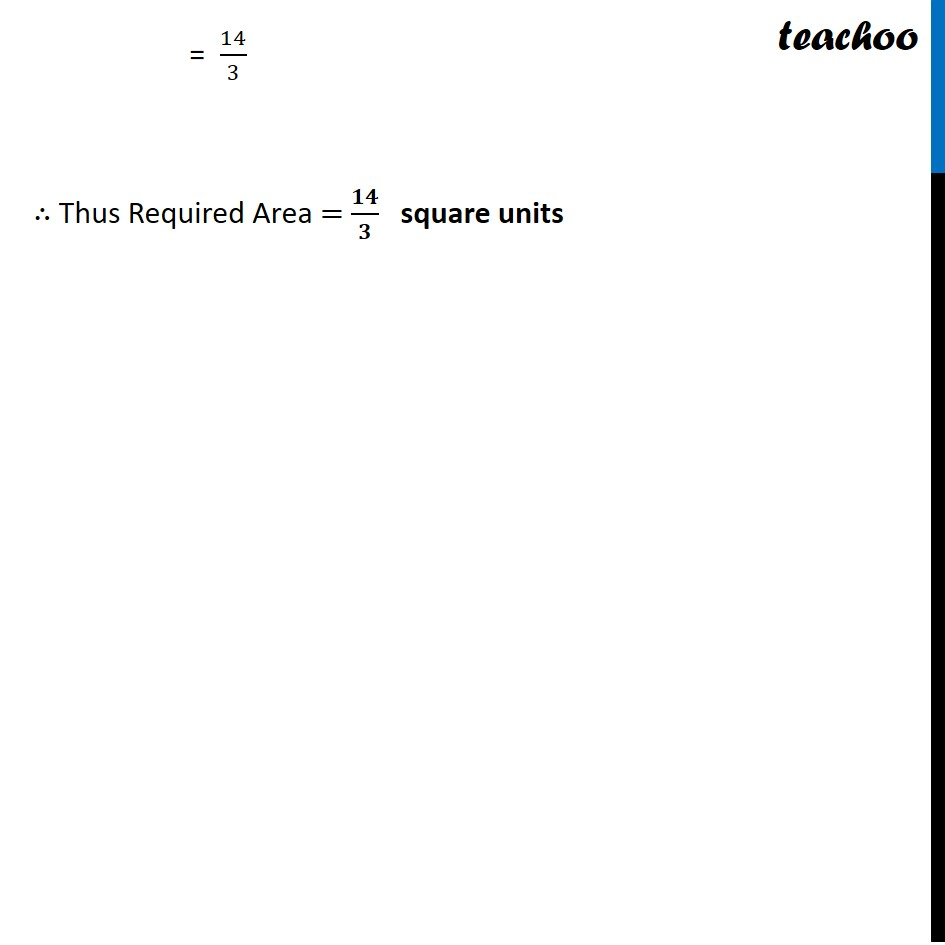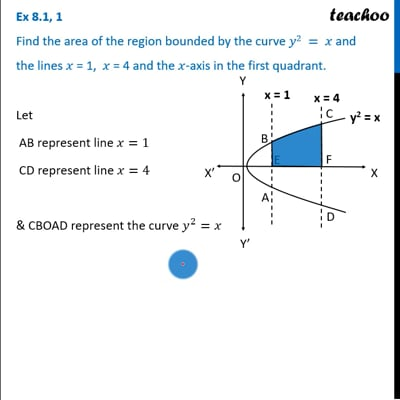This video is only available for Teachoo black users

Introducing your new favourite teacher - Teachoo Black, at only ₹83 per month

### Transcript

Ex 8.1, 1 Find the area of the region bounded by the curve 𝑦2 = 𝑥 and the lines 𝑥 = 1, 𝑥 = 4 and the 𝑥-axis in ﻿the first quadrant.Let AB represent line 𝑥=1 CD represent line 𝑥=4 & CBOAD represent the curve 𝑦^2=𝑥 Since we need area in the first quadrant We have to find area of BCFE Area of BCFE = ∫_𝟏^𝟒▒𝒚 . 𝒅𝒙 So, we need to calculate ∫_𝟏^𝟒▒𝒚 . 𝒅𝒙 We know that 𝑦^2=𝑥 Taking square root on both sides ∴ 𝑦=±√𝑥 Since BCEF is in 1st Quadrant ∴ 𝒚=√𝒙 Area of BCFE = ∫_1^4▒𝑦 . 𝑑𝑥 = ∫_𝟏^𝟒▒√𝒙 . 𝒅𝒙 = ∫_1^4▒〖(𝑥)^(1/2) 𝑑𝑥〗 = [𝑥^(1/2+1)/(1/2 +1)]_1^4 = [ 𝑥^(3/2)/(3/2) ]_1^4 = 𝟐/𝟑 [𝒙^(𝟑/𝟐) ]_𝟏^𝟒 = 2/3 {(4)^(3/2)−(1)^(3/2) } = 2/3 {[(4)^(1/2) ]^3−1} = 𝟐/𝟑 {(𝟐)^𝟑−𝟏} = 2/3 [8−1] = 2/3 × 7 = 14/3 ∴ Thus Required Area = 𝟏𝟒/𝟑 square units